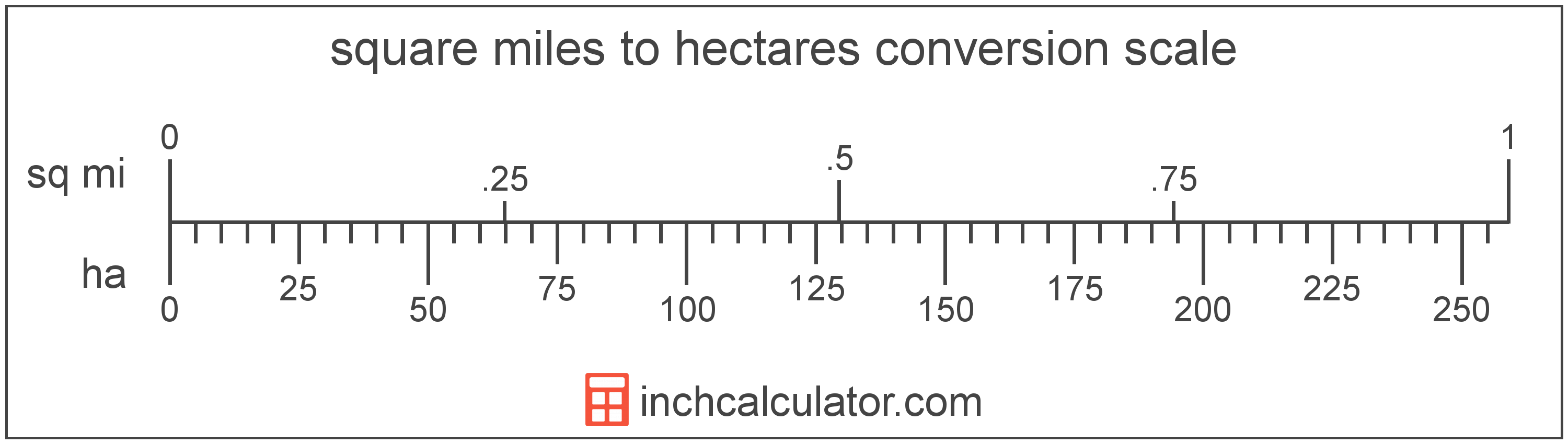# Square Miles to Hectares Converter

Enter the area in square miles below to get the value converted to hectares.

Results in Hectares:1 sq mi = 258.998811 ha

Do you want to convert hectares to square miles?

## How to Convert Square Miles to Hectares

To convert a measurement in square miles to a measurement in hectares, multiply the area by the following conversion ratio: 258.998811 hectares/square mile.

Since one square mile is equal to 258.998811 hectares, you can use this simple formula to convert:

hectares = square miles × 258.998811

The area in hectares is equal to the area in square miles multiplied by 258.998811.

For example, here's how to convert 5 square miles to hectares using the formula above.
hectares = (5 sq mi × 258.998811) = 1,294.994055 ha### How Many Hectares Are in a Square Mile?

There are 258.998811 hectares in a square mile, which is why we use this value in the formula above.

1 sq mi = 258.998811 ha

## What is a Square Mile?

One square mile is equal to the area of a square with sides that are each 1 mile long. One square mile is roughly equal to 2.59 square kilometers or 640 acres.

The square mile is a US customary and imperial unit of area. Square miles can be abbreviated as sq mi, and are also sometimes abbreviated as mi². For example, 1 square mile can be written as 1 sq mi or 1 mi².

## What is a Hectare?

One hectare is equal to 10,000 square meters, or the area of a square with 100 meter sides.

The hectare is an SI accepted unit for area for use with the metric system. In the metric system, "hecto" is the prefix for 102. Hectares can be abbreviated as ha; for example, 1 hectare can be written as 1 ha.

## Square Mile to Hectare Conversion Table

Table showing various square mile measurements converted to hectares.
Square Miles Hectares
1 sq mi 259 ha
2 sq mi 518 ha
3 sq mi 777 ha
4 sq mi 1,036 ha
5 sq mi 1,295 ha
6 sq mi 1,554 ha
7 sq mi 1,813 ha
8 sq mi 2,072 ha
9 sq mi 2,331 ha
10 sq mi 2,590 ha
11 sq mi 2,849 ha
12 sq mi 3,108 ha
13 sq mi 3,367 ha
14 sq mi 3,626 ha
15 sq mi 3,885 ha
16 sq mi 4,144 ha
17 sq mi 4,403 ha
18 sq mi 4,662 ha
19 sq mi 4,921 ha
20 sq mi 5,180 ha
21 sq mi 5,439 ha
22 sq mi 5,698 ha
23 sq mi 5,957 ha
24 sq mi 6,216 ha
25 sq mi 6,475 ha
26 sq mi 6,734 ha
27 sq mi 6,993 ha
28 sq mi 7,252 ha
29 sq mi 7,511 ha
30 sq mi 7,770 ha
31 sq mi 8,029 ha
32 sq mi 8,288 ha
33 sq mi 8,547 ha
34 sq mi 8,806 ha
35 sq mi 9,065 ha
36 sq mi 9,324 ha
37 sq mi 9,583 ha
38 sq mi 9,842 ha
39 sq mi 10,101 ha
40 sq mi 10,360 ha

## References

1. Cambridge Dictionary, hectare, https://dictionary.cambridge.org/us/dictionary/english/hectare